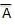# NCERT Solutions Class 12 Computer Science Boolean Algebra

Boolean Algebra used to simplify and analyze the digital logic circuits. It is the branch of algebra in which the values of the variables are the truth values true and false. The true value is represented by 1 and the false value is represented by 0.

## Boolean Laws

Commutative Law

i) A + B = B + A

ii) A . B = B . A

Associative Law

i) (A . B) . C = A . (B . C)

ii) (A+B) + C = A + (B + C)

Distributive Law

i) A . (B + C) = A . B + A . C

AND Law

i) A . 0 = 0

ii) A . 1 = A

iii) A . A = AOR Law

i) A + 0 = A

ii) A + 1 = 1

iii) A + A = ABoolean Rules

Only two values can we be used for variables. Binary 0 for Low and Binary 1 for High.

The complement of a variable is represented by a bar above it (-). The complement of A is denoted as.